TitleCollege Algebra
Answer/Discussion to Practice Problems
Tutorial 23B: Rational InequalitiesAnswer/Discussion to 1a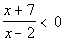I used the test-point method, but you could also use the sign graph of factors method.

 Step 1: Write the rational inequality in standard form.

 This quadratic inequality is already in standard form.

 Step 2: Factor the numerator and denominator and find the values of x that make these factors equal to 0 to find the boundary points.

 Numerator: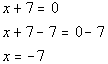*Set numerator = 0 and solve

 Denominator: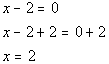*Set denominator = 0 and solve

 -7 and 2 are boundary points.

 Below is a graph that marks off the boundary points -7 and 2 and shows the three sections that those points have created on the graph.  Note that open holes were used on those two points since our original inequality did not include where it is equal to 0 and 2 makes the denominator 0.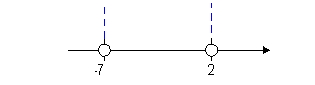Note that the two boundary points create three sections on the graph: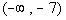,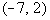, and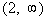.

 Step 4: Test a point in each test interval found in step 3 to see which interval(s) is part of the solution set.

 You can choose ANY point in an interval to represent that interval.  Remember that we are not interested in the actual value that we get, but what SIGN (positive or negative) that we get. Keep in mind that our original problem is.  Since we are looking for the quadratic expression to be LESS THAN 0, that means we need our sign to be NEGATIVE. From the interval, I choose to use -8 to test this interval: (I could have used -10, -25, or -10000 as long as it is in the interval)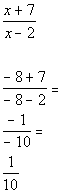*Chose -8 from 1st interval to plug in for x

 Since 1/10 is positive and we are looking for values that cause our quadratic expression to be less than 0 (negative),would not be part of the solution.

 From the interval, I choose to use 0 to test this interval. (I could have used -6, -5, or -4 as long as it is in the interval)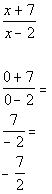*Chose 0 from 2nd interval to plug in for x

 Since -7/2 is negative and we are looking for values that cause our expression to be less than 0 (negative),would be part of the solution.

 From the interval, I choose to use 3 to test this interval. (I could have used 10, 25, or 10000 as long as it is in the interval)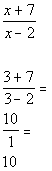*Chose 3 from 3rd interval to plug in for x

 Since 10 is positive and we are looking for values that cause our quadratic expression to be less than 0 (negative),would not be part of the solution.

 Interval notation:Graph: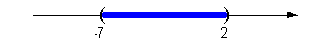*Open interval indicating all values between -7 and 2 *Visual showing all numbers between -7 and 2 on the number lineAnswer/Discussion to 1b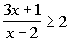I used the test-point method, but you could also use the sign graph of factors method.

 Step 1: Write the rational inequality in standard form.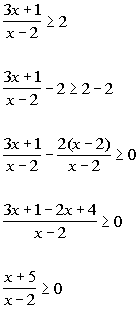*Inv. of add. 2 is sub. 2     *Rewrite 2nd fraction with LCD of x - 2

 Step 2: Factor the numerator and denominator and find the values of x that make these factors equal to 0 to find the boundary points.

 Numerator: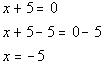*Set numerator = 0 and solve

 Denominator:*Set denominator = 0 and solve

 -5 and 2 are boundary points.

 Below is a graph that marks off the boundary points -5 and 2 and shows the three sections that those points have created on the graph.  Note that there is an open hole at -2.  Since that is the value that cause the denominator to be 0, we cannot include where x = 2.  Since our inequality includes where it is equal to 0, and -5 causes only the numerator to be 0 there is a closed hole at -5.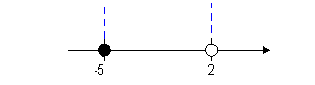Note that the two boundary points create three sections on the graph: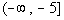,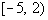, and.

 Step 4: Test a point in each test interval found in step 3 to see which interval(s) is part of the solution set.

 You can choose ANY point in an interval to represent that interval.  Remember that we are not interested in the actual value that we get, but what SIGN (positive or negative) that we get. Keep in mind that our inequality is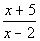> 0.  Since we are looking for the quadratic expression to be GREATER THAN OR EQUAL TO 0, that means we need our sign to be POSITIVE (OR O). From the interval, I choose to use -6 to test this interval: (I could have used -10, -25, or -10000 as long as it is in the interval)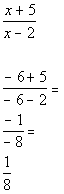*Chose -6 from 1st interval to plug in for x

 Since 1/8 is positive and we are looking for values that cause our quadratic expression to be greater than or equal to 0 (positive or 0),would be part of the solution.

 From the interval, I choose to use 0 to test this interval. (I could have used -4, -3, or -2 as long as it is in the interval)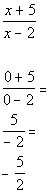*Chose 0 from 2nd interval to plug in for x

 Since -5/2 is negative and we are looking for values that cause our expression to be greater than or equal to 0 (positive or 0),would not be part of the solution.

 From the interval, I choose to use 3 to test this interval. (I could have used 10, 25, or 10000 as long as it is in the interval)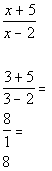*Chose 3 from 3rd interval to plug in for x

 Since 8 is positive and we are looking for values that cause our quadratic expression to be greater than or equal to 0 (positive or 0),would be part of the solution.

 Interval notation: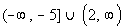Graph: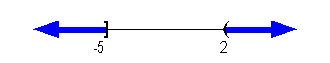*An closed interval indicating all values less than or equal to -5  and an open  interval indicating all values greater then 2 *Visual showing all numbers less than or equal to -5  and indicating all values greater then 2

Last revised on Jan. 2, 2010 by Kim Seward.
All contents copyright (C) 2002 - 2010, WTAMU and Kim Seward. All rights reserved.